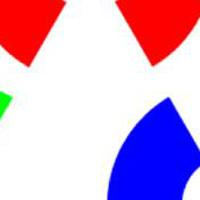## Basics

Creating a 2D matrix is very simple. You use the cvCreateMat function. It has the following prototype. The rows _and _cols _parameters are self explanatory. And to create a 1D matrix, you set either _cols=1 (a column matrix) or rows=1 (a row matrix).

The type parameter lets you choose from the large variety of formats. There's a large number of predefined constants to help you remember. Their general form is: CV_(S|U|F)C.

```CvMat* cvCreateMat (int rows, int cols, int type);
```
• Bit depth can be 8, 16, 32 or 64 bits. This is the amount of memory for the single orange cell in the above picture.
• S means signed integer, U means unsigned integer and F means floating point (decimal values)
• You can have any number of channels

Thus, CV_8UC3 is for 8 bit unsigned integer matrices with 3 channels. CV_32SC2 is for 32 bit signed integer matrices with 2 channels. CV_64FC1 is for 64 bit floating point matrices with a single channel.

Internally, the CvMat structure looks like this:

```typedef struct CvMat
{
int type;
int step;

int* refcount; /* underlying data reference counter */

union
{
uchar* ptr;
short* s;
int* i;
float* fl;
double* db;

} data;

int rows;
int cols;
} CvMat;
```
• type is the same parameter _type _ORed with some more constants
• step is the size of a row in bytes
• refcount is for internal use only
• data is used to access elements of the matrix (we'll get to it in a minute)
• rows is the number of rows
• cols is the number of columns

## More functions

The cvCreateMat function is equivalent to calling cvCreateMatHeader, and then calling cvCreateData. The "header" is the structure I just talked about, and the "data" is the actual memory where the matrix is stored. So, you can create matrix and not allocate data. That's a really handy feature. It really puts you in control of managing memory through the program.

Once you've created a matrix, you can release it using the cvReleaseMat function. It's important that you release whatever memory you allocate. Or you'll end up with a program that leaks a lot of memory. Not good.

Another utility function is cvCloneMat. This function creates an exact copy of the matrix you supply. It duplicate the contents in memory, so both copies are independent of each other.

Here are the function prototypes for each of them:

```CvMat* cvCreateMatHeader(int rows, int cols, int type)
void cvCreateData(CvMat*)
void cvReleaseMat(CvMat**)
CvMat* cvCloneMat(CvMat*)
```

## Accessing data

Well, you've made a matrix. Now you better know how to access whats in it! Here are some functions to do that:

```double cvGetReal1D(CvArr* arr, int idx0)
double cvGetReal2D(CvArr* arr, int idx0, int idx1)
double cvGetReal3D(CvArr* arr, int idx0, int idx1, int idx2)
double cvGetRealND(CvArr* arr, int* idx)
```

Simple as that. You supply the indices, and you get the value at a particular element for a 1D, 2D, 3D or an N-dimensional matrix.

To set values in the matrix, you use a similar group of functions:

```void cvSetReal1D(CvArr* arr, int idx0, double value)
void cvSetReal2D(CvArr* arr, int idx0, int idx1, double value)
void cvSetReal3D(CvArr* arr, int idx0, int idx1, int idx2, double value)
void cvSetRealND(CvArr* arr, int* idx, double value)
```

Yet another way of accessing data is using pointers. And, pointers are definitely more efficient than the functions above. The idea is to get the pointer to a particular location in the matrix, and then use pointer arithmetic to move around the matrix.

```uchar* cvPtr1D(CvArr* arr, int idx0)
uchar* cvPtr2D(CvArr* arr, int idx0, int idx1)
uchar* cvPtr3D(CvArr* arr, int idx0, int idx1, int idx2)
uchar* cvPtrND(CvArr* arr, int idx)
```

With these you get the pointer. Do whatever you want. Set values, retrieve values, whatever!

Note that the parameters are CvArr _pointers. So you can pass _CvMat variables and also _IplImage _variables. You can check the reason for this in the article Primitive structures in OpenCV.

## Caution with pointers

Here are two things you need to take care of when using the cvPtr*D functions.

Warning #1: Channels are stored interleaved. That is, if you have a 3 channel matrix, say representing the RGB components of an image, then it will be stored as rgbrgbrgb. Make sure you take that into account when doing the pointer arithmetic.

Warning #2: Use the step data member. It stores the actual number of bytes taken up by a row. For processor efficiency reasons, the memory allocated is increased to the nearest 4 byte boundary. So, if the row width for a particular matrix is 33 bytes, it will be increased to 36 bytes. And step stores this "total" memory allocated to the row. So use it to move through rows.

#### Related posts#### Efficiently accessing matrices#### Mathematical Morphology in OpenCV

Utkarsh Sinha created AI Shack in 2010 and has since been working on computer vision and related fields. He is currently at Microsoft working on computer vision.